## Friday, June 27, 2008

### Irodov Problem 1.188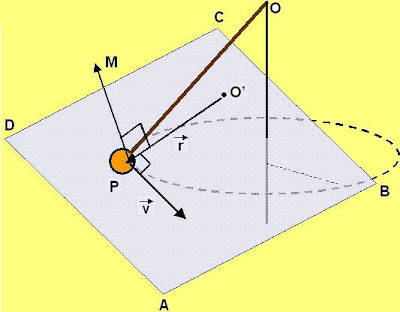Suppose that that particle is located at a location P moving along a circular path as shown in the figure. Suppose that the observer is located at point O'. Suppose that the particle is moving with velocity v and the length of O'P is r. The angular momentum of the particle as seen from O' will be,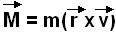Since the magnitude of velocity v is constant throughout the particles rotation along its circular path, in order for the magnitude of angular momentum M to be constant, the magnitude of r must be constant. This means that the length O'P should not change as the particle moves along the circle. This is possible only of O' lies on the axis of rotation of the particle.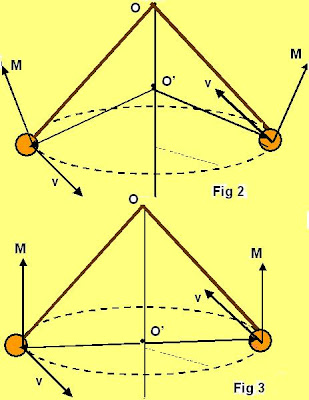Now let us consider what happens if O' lies at some arbitrary point on the axis. The vector M will be perpendicular to the plane ABCD containing the vectors r and v as shown in the first figure. In Fig2 we consider a certain point O' that lies on the axis of rotation but is above the plane of rotation of the particle. While the magnitude of the angular momentum will be the same as the particle rotates along the circle, its direction will not be the same. In Figure 2 the direction of M is shown for two different positions of the particle along its path to illustrate how the direction on M changes.

Only when O' is on the same plane as the plane of rotation of the particle, the direction of M will always remain the same (parallel to the axis of rotation). Thus, for the both the direction and magnitude of M to be the same as the particle moves along its path, O' must lie at th center of the circle as shown in figure 3. Hence there is only one point from where the angular momentum of the particle will not change and that is the center of the circle.

The above fact can also be rigorously mathematically derived but I intentionally refrained from the arduous exercise.

b)Suppose that the string holding the particle is slanted at an angle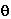to the vertical. This means that the radius of the circle along which the particle rotates is. The velocity of the particle will thus be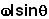directed along the tangent of the circle as shown in the figure. Let the tension in the string be T. There are two forces acting on the mass in the vertical direction i) the force of gravity mg and ii) the component of tension. There is only one force acting in the horizontal direction, the component of tension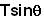. Due to its rotation in a circle, the particle experiences a centripetal acceleration ofalong the radial direction as shown in the figure. So we have,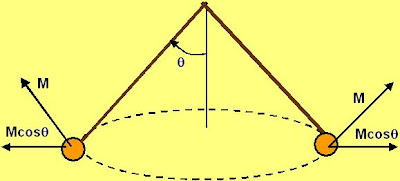The magnitude of angular momentum vector will not change as the particle moves along the circle however its direction will as shown in the figure. Also the vertical component of the angular momentum will remain changes all through its motion, only its horizontal component will be rotating so that its always aligned radially outwards. So by the time the particle rotates half a circle its net change in momentum will be